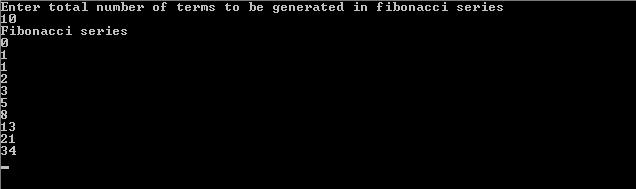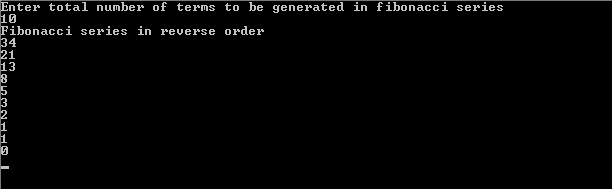# C Program for Fibonacci Series

C Program for Fibonacci Series using for loop, recursion and array along with the output is given below.

## About Fibonacci Series

The Fibonacci series or Fibonacci numbers or Fibonacci sequence always consists of 0 and 1 as its first two numbers with each subsequent number in the series as the sum of the previous two numbers.

Mathematically, the sequence Fn of Fibonacci numbers is defined as

F0= 0, F1 = 1

Fn = Fn-1 + Fn-2

For example, the following numbers are in fibonacci series:

0, 1, 1, 2, 3, 5, 8, 13, 21, 34, 55, 89, 144, 233, 377, ….

## C Program for Fibonacci Series Using Loop

```#include<stdio.h>

void main()
{
int n, i, a=0, b=1, next;

printf("Enter total number of terms to be generated in fibonacci seriesn");
scanf("%d", &n);
printf("Fibonacci seriesn");
for(i=0; i<n; i++)
{
if(i<=1)
next= i;
else
{
next=a+b;
a=b;
b=next;
}
printf("%dn",next);

}
getch();
}

```

### Output## C Program for Fibonacci Series Using Recursion

```#include<stdio.h>

void main()
{
int n, i;
printf("Enter total number of terms to be generated in fibonacci seriesn");
scanf("%d", &n);
printf("Fibonacci seriesn");
for(i=0; i<n; i++)
printf("%dn",fibonacci(i));
getch();
}
int fibonacci(int i)
{
if(i==0 || i==1)
return i;
return (fibonacci(i-1)+fibonacci(i-2));

}

```

## C Program for Fibonacci Series Using Array

```#include<stdio.h>

void main()
{
int n, a, i;
printf("Enter total number of terms to be generated in fibonacci seriesn");
scanf("%d", &n);
for(i=0; i<n; i++)
a[i]=fibonacci(i);

printf("Fibonacci seriesn");

for(i=0; i<n; i++)
printf("%dn",a[i]);
getch();
}
int fibonacci(int i)
{
if(i==0 || i==1)
return i;
return (fibonacci(i-1)+fibonacci(i-2));

}
```

## C Program for Fibonacci Series in Reverse Order Using Array

```#include<stdio.h>

void main()
{
int n, a, i;
printf("Enter total number of terms to be generated in fibonacci seriesn");
scanf("%d", &n);
for(i=0; i<n; i++)
a[i]=fibonacci(i);

printf("Fibonacci series in reverse ordern");

for(i=n-1; i>=0; i--)
printf("%dn",a[i]);
getch();
}
int fibonacci(int i)
{
if(i==0 || i==1)
return i;
return (fibonacci(i-1)+fibonacci(i-2));

}
```

### OutputPlease comment with your real name using good manners.

This site uses Akismet to reduce spam. Learn how your comment data is processed.